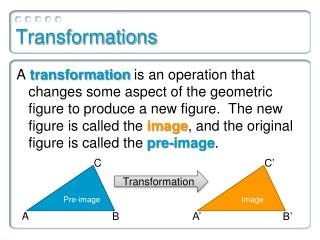Download PresentationTransformations

# Transformations - PowerPoint PPT Presentation

Transformations. A transformation is an operation that changes some aspect of the geometric figure to produce a new figure. The new figure is called the image , and the original figure is called the pre-image. C. C’. Pre-image. Image. Transformation. A. A’. B. B’.I am the owner, or an agent authorized to act on behalf of the owner, of the copyrighted work described.
Download Presentation## Transformations

Download Policy: Content on the Website is provided to you AS IS for your information and personal use and may not be sold / licensed / shared on other websites without getting consent from its author.While downloading, if for some reason you are not able to download a presentation, the publisher may have deleted the file from their server.

- - - - - - - - - - - - - - - - - - - - - - - - - - E N D - - - - - - - - - - - - - - - - - - - - - - - - - -
Presentation Transcript
1. Transformations A transformationis an operation that changes some aspect of the geometric figure to produce a new figure. The new figure is called the image, and the original figure is called the pre-image. C C’ Pre-image Image Transformation A A’ B B’

2. Congruence Transformations A congruence transformation, or isometry, is a type of transformation that changes the position of a figure without changing its size or shape. • In other words, in an isometry, the pre-image is congruent to the image. • There are three basic isometries…

3. Isometries Which of the following transformations is not an isometry?

4. Tessellations An interesting application of transformations is a tessellation. A tessellation is a tiling of a plane with one or more shapes with no gaps or overlaps. They can be created using transformations.

5. Tessellations

6. Tessellations

7. Vectors Translations are usually done with a vector, which gives a direction and distance to move our shape.

8. Vectors Translations are usually done with a vector, which gives a direction and distance to move our shape.

9. Transformation Coordinate Rules What are the new coordinates of the point (x, y) under each of the following transformations? • Translation under the vector a, b • Reflection across the x-axis Reflection across the y-axis • Reflection across the line y= x Reflection across the line y = -x • Rotation of 90° around the origin

10. Transformation Coordinate Rules Coordinate Notation for a Translation You can describe a translation of the point (x, y) under the vector a, b by the notation:

11. Transformation Coordinate Rules Coordinate Notation for a Reflection

12. Transformation Coordinate Rules Coordinate Notation for a Rotation

13. Example 1 Draw and label ABC after each of the following transformations: • Reflection across the x-axis • Reflection across the y-axis • Translation under the vector -3, 5

14. Example 2 What translation vector was used to translate ABC to A’B’C’? Write a coordinate rule for the translation. Vector: a, b = 10, -2 Rule: (x, y)  (x + 10, y – 2)

15. Example 3 Draw the image of ABC after it has been rotated 90° counterclockwise around the origin.

16. Example 3 Draw the image of ABC after it has been rotated 90° counterclockwise around the origin.

17. Example 4a Does the order matter when you perform multiple transformations in a row?

18. Example 4b Does the order matter when you perform multiple transformations in a row?

19. Example 4c Does the order matter when you perform multiple transformations in a row?

20. Composition of Transformations Two or more transformations can be combined to make a single transformation called a composition of transformations.

21. Composition of Transformations When the transformations being composed are of different types (like a translation followed by a reflection), then the order of the transformations is usually important.

22. Glide Reflection A special type of composition of transformations starts with a translation followed by a reflection. This is called a glide reflection.

23. Glide Reflection A special type of composition of transformations starts with a translation followed by a reflection. This is called a glide reflection.